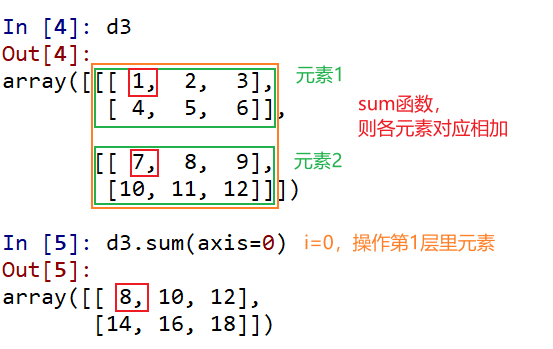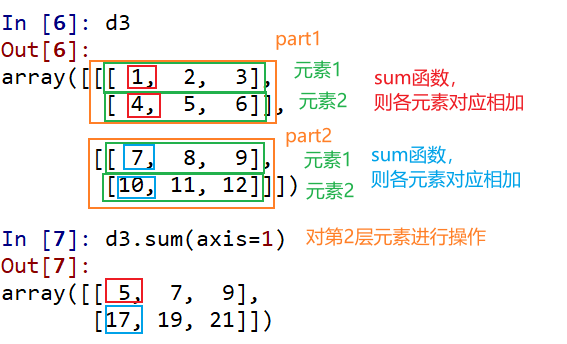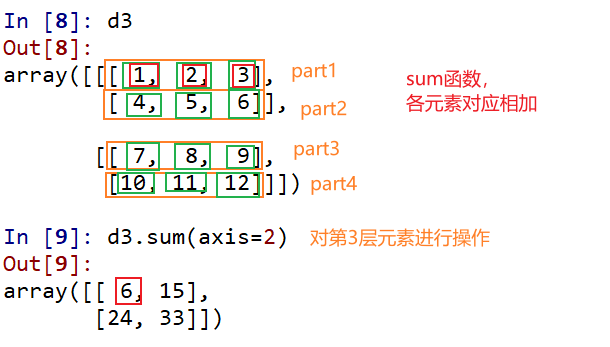# Numpy中axis参数的理解

1. Numpy 中 axis=i 中的 i 代表的轴不固定，不同维度编排不同，可以把它看成是种由外向内的编排方式
2. Numpy 中 axis=i 中的 i 表示数组索引的第 i+1 层
3. axis=i 即沿着 i 轴方向进行相应操作
4. axis=i 即对索引的第 i+1 层元素进行相应操作

——《道德经》

 ``````1 2 3 4 5 6 `````` ``````In : import numpy as np In : d1 = np.array([1,2,3]) In : d1 Out: array([1, 2, 3]) ````````````1 2 3 4 5 6 `````` ``````In : d2 = np.array([[1,2,3],[4,5,6]]) In : d2 Out: array([[1, 2, 3], [4, 5, 6]]) ``````

1.Numpy 中 axis=i 中的 i 代表的轴不固定，不同维度编排不同，可以把它看成是种由外向内的编排方式``````1 2 3 4 5 6 7 8 9 `````` ``````In : d3 = np.array([[[1,2,3],[4,5,6]],[[7,8,9],[10,11,12]]]) In : d3 Out: array([[[ 1, 2, 3], [ 4, 5, 6]], [[ 7, 8, 9], [10, 11, 12]]]) ``````2.Numpy 中 axis=i 中的 i 表示数组索引的第 i+1 层`````` 1 2 3 4 5 6 7 8 9 10 11 12 `````` ``````In : d3 Out: array([[[ 1, 2, 3], [ 4, 5, 6]], [[ 7, 8, 9], [10, 11, 12]]]) In : d3 Out: array([[ 7, 8, 9], [10, 11, 12]]) ``````

3.axis=i 即沿着 i 轴方向进行相应操作

 ``````1 2 3 4 5 6 7 `````` ``````In : d2 Out: array([[1, 2, 3], [4, 5, 6]]) In : d2.sum(axis=0) Out: array([5, 7, 9]) ```````````` 1 2 3 4 5 6 7 8 9 10 11 12 `````` ``````In : d3 Out: array([[[ 1, 2, 3], [ 4, 5, 6]], [[ 7, 8, 9], [10, 11, 12]]]) In : d3.sum(axis=0) Out: array([[ 8, 10, 12], [14, 16, 18]]) ```````````` 1 2 3 4 5 6 7 8 9 10 11 12 `````` ``````In : d3 Out: array([[[ 1, 2, 3], [ 4, 5, 6]], [[ 7, 8, 9], [10, 11, 12]]]) In : d3.sum(axis=1) Out: array([[ 5, 7, 9], [17, 19, 21]]) ``````axis=2 同理。

4.axis=i 即对索引的第 i+1 层元素进行相应操作axis=1 就是对第二层索引元素进行对应操作。axis=2 就是对第三层索引元素进行对应操作。